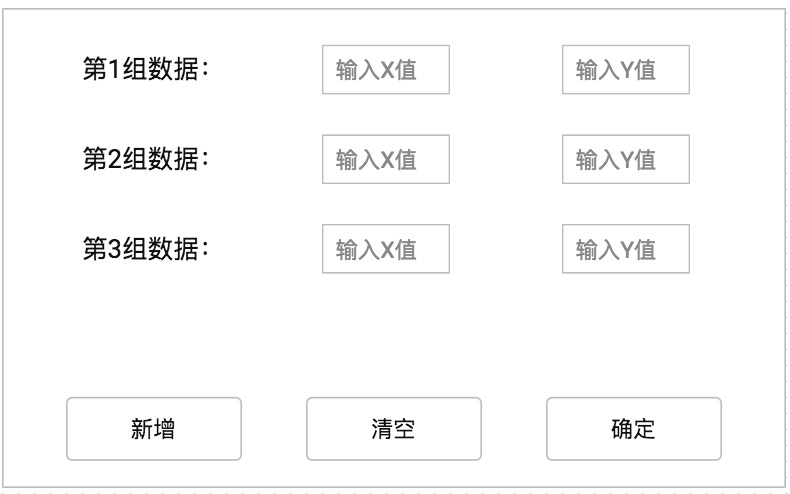from tkinter import *
root = Tk()
root.title("元素周期表")
def cal():
master = Tk()
v1=StringVar()
v2=StringVar()
v3=StringVar()
frame = Frame(master)
Entry(frame,width=10,textvariable=v1).grid(row = 0, column = 1)
Entry(frame,width=10,textvariable=v2).grid(row = 1, column = 1)
Entry(frame,width=10,textvariable=v3).grid(row = 2, column = 1)
Button(frame,text="数1").grid(row=0,column=0)
Button(frame,text="数2").grid(row=1,column=0)
Button(frame,text="数3").grid(row=2,column=0)

Button(root,text="选择",command=cal).grid(row=2,column=1)
Button(root,text="输入一个数",command=cal).grid(row=2,column=1)
Button(root,text="输入另一个数",command=cal).grid(row=2,column=2)

mainloop()

3个回答

`````` from tkinter import *

root = Tk()
root.title("元素周期表")

def cal(type):
master = Tk()
v1 = StringVar()
v2 = StringVar()
v3 = StringVar()
frame = Frame(master)

Entry(frame, width=10, textvariable=v1).grid(row=0, column=1)
Button(frame, text="数1").grid(row=0, column=0)

if (type == 1): return;
Entry(frame, width=10, textvariable=v2).grid(row=1, column=1)
Button(frame, text="数2").grid(row=1, column=0)

if (type == 2): return;
Entry(frame, width=10, textvariable=v3).grid(row=2, column=1)
Button(frame, text="数3").grid(row=2, column=0)

Button(root, text="选择", command=cal).grid(row=2, column=1)
Button(root, text="输入一个数", command=lambda :cal(1)).grid(row=2, column=1)
Button(root, text="输入另一个数", command=lambda :cal(2)).grid(row=2, column=2)

mainloop()
``````fanshinee 回复caozhy: 您好，没有 办法传图，您看下我回复的那个fanshinee 老师您好，使用tkinter中的button和enry按钮，增加输入框（通过），这些输入框怎么能够有序的排列，并且按照某种运算（例如加法）运算？fanshinee 您好，非常感谢您的回复，可是我刚刚测试了下，您这个每次点击一下都会出现一个新的小窗口，我希望每次都出现在一个小窗口。js选择一个选项 跳出另一个选项 跳出一个输入框

最终的运行效果图（程序见序号4）   #!/usr/bin/env python # -*- coding:utf-8 -*- # ------------------------------------------------------------ #!/usr/bin/env python # -*- coding:utf-8 -*- # ---------------...
android弹出消息框、输入框、选择框、日期选择

MouseListener pmUnCreate = new MouseAdapter()rn public void mouseClicked(MouseEvent ex)rn rn JPopupMenu ppopUnCreate= new JPopupMenu();rn ppopUnCreate.setVisible(false);rn JMenuItem pjMen = null;rn if ( psCellsU[piSelLine].equals("桳") )rn rn Vector pvPatTempRep = (Vector)phReptypnm.get(rn psCellsU[piSelLine]+psCellsU[piSelLine]);rn for ( int i = 0 ; i < pvPatTempRep.size() ; i ++ )rn rn pjMen = new JMenuItem(PSNULL + pvPatTempRep.get(i));rn pjMen.setBackground(Color.white);rn pjMen.setForeground(Color.black);rn pjMen.setEnabled(false);rn ppopUnCreate.add(pjMen);rn rn ppopUnCreate.setBorder(BorderFactory.createLineBorder(Color.black));rn if ( piSelcol == PICELLLINES.length - 3 &&rn pvPatTempRep.size() != 0 )rn rn ppopUnCreate.show(ex.getComponent(),ex.getX()+10,ex.getY()+10);rn rn rn rn;

Python tkinter实现先弹出选择框后弹出输入框，并得到输入框的值
Python 自带的GUI tkinter实现界面编写有点难，这次我遇到的问题如下所示：当我一开始进入系统时弹出如下选择框，我点击确定即可弹出输入框，可当我输入好相应信息之后点击确定，却拿不到我在输入框输入的值。这个情况的具体代码如下：import tkinter as tk from tkinter import messagebox def on_click(): global U...

PopupCalendar日历选择输入框
PopupCalendar日历选择输入框,日历选择框，选择输入。很好用的，希望大家下载。

Title             *{             margin: 0;             padding: 0;         }         ul{             width: 400px;             height: 400px;             border:1px solid #999;         }

&amp;lt;%@ taglib prefix=&quot;c&quot;   uri=&quot;http://java.sun.com/jsp/jstl/core&quot; %&amp;gt; &amp;lt;!DOCTYPE html PUBLIC &quot;-//W3C//DTD HTML 4.01 Transitional//EN&quot; &quot;http://www.w3.org/TR/html4/loose.dtd&quot;&amp;gt;  &amp;lt;%--项目路径 -
input输入框选择美化

TDBedit 的输入框的默认值在选择后默认值消失。。。。。
TDBedit 的输入框的默认值在鼠标选择后默认值消失。。。。。在什么地方改成不消失的。。。。rnTDBedit中只有这个事件rnprocedure TCustomManager.ThighsizeKeyPress(Sender: TObject; var Key: Char);rnbeginrn if Key = #13 thenrn beginrn Calfsize.SetFocus;rn end;rnend;

RT
jeecg如何在一个页面使用按钮选择弹出选择框
1.在页面需要的地方加上如下代码: id="userId" name="userId" type="hidden" style="width: 150px" class="inputxt" ignore="ignore"/> name="userName" class="inputxt" value="" id="userName" readonly="readonly" datatype=

function delAllSelectOption(SelectName,win)rn //设置Select选择框的值，win是窗口对象rn if(win==null)win=windowrn with(win)rn rn var Selectobj = eval("document.forms."+SelectName);rn for(var i=Selectobj.options.length;i>0;i--)rn Selectobj.options.remove(i-1)rn rnrnrn如题，这个函数要在Mozilla下如何调整？remove函数貌似只能在IE下运行。
jquery选择select的选项

raid选择启动盘选项
-